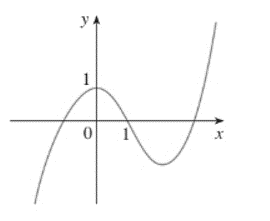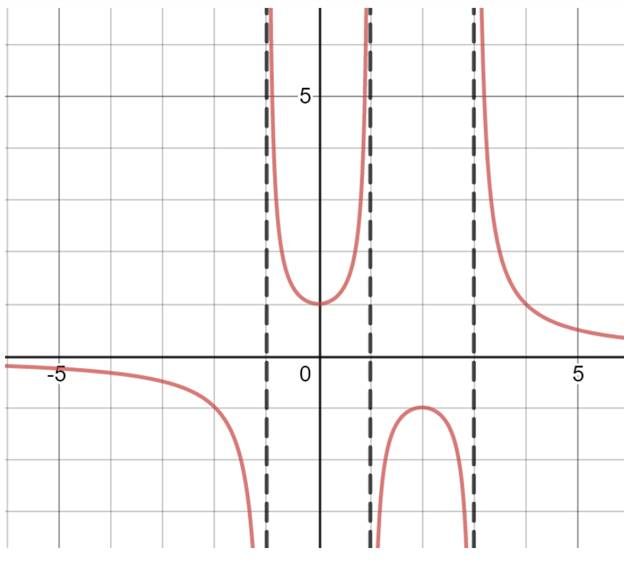# graph of y = 1 / f ( x ) by using the graph and explain the features of f are the most important.### Single Variable Calculus: Concepts...

4th Edition
James Stewart
Publisher: Cengage Learning
ISBN: 9781337687805### Single Variable Calculus: Concepts...

4th Edition
James Stewart
Publisher: Cengage Learning
ISBN: 9781337687805

#### Solutions

Chapter 1.3, Problem 28E
To determine

## To sketch:graph of y=1/f(x) by using the graph and explain the features of f are the most important.

Expert Solution

1. Wipe the part of graph which is to the left of y -axis.
2. Now reflect the right part of graph in y -axis.

### Explanation of Solution

Given:

The given graph is:Calculation:

As it isnoticed a couple of things. First, function f has zeros at x=1,x=1, and x=3 .

This means that y=1/f(x) will not defined in those points. The function will have vertical asymptotes at these points.

Next, where f(x) is increasing on 1/f(x) and f(x) is decreasing. Also, where f(x) is decreasing, 1/f(x) is increasing,

So y=1/f(x) increasing on (2,2) and decreasing on (,0) and (2,) .

Also, where f(x) is positive, 1/f(x) is positive as well. And where f(x) is negative, 1/f(x) will be negative.

Now, it is enough information to draw the graph. First, draw asymptote and then use interval of monotonicity and signs to plot a graph.

The graph is:### Have a homework question?

Subscribe to bartleby learn! Ask subject matter experts 30 homework questions each month. Plus, you’ll have access to millions of step-by-step textbook answers!